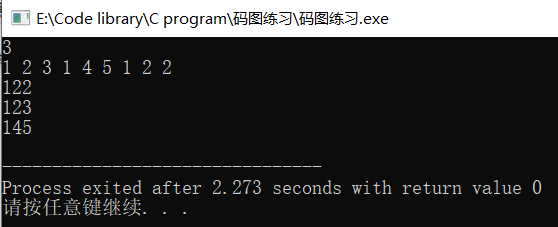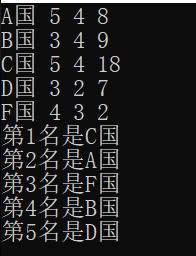• 2019-11-29 16:40:29

## 对结构体数组进行排序

题目：对结构体数组进行排序。

题目描述：
struct Person{
int no;
int age;
int height;
}
实现sort方法对结构体数组进行排序。
void sort(Person * array,int n);

根据no从小到大排序；如果no相同则根据age排序；如果age相同，则根据height排序。

注意：
遇到异常情况，输出"error"；否则不要随意输出，会视为错误。

代码：
#include <stdio.h>
#include <string.h>

struct Person{
int no;
int age;
int height;
}temp;

void sort(struct Person * array,int n)
{
int i = 0;
int j = 0;
if(n > 0 && array != NULL)
{
for (i = 0; i < n - 1; i++)
{
for (j = 0; j < n - 1 -i; j++)
{
if (array[j].no > array[j+1].no)
{
temp = array[j];
array[j] = array[j+1];
array[j+1] = temp;
}
else if (array[j].no == array[j+1].no && array[j].age > array[j+1].age)
{
temp = array[j];
array[j] = array[j+1];
array[j+1] = temp;
}
else if ((array[j].no == array[j+1].no) && (array[j].age == array[j+1].age)
&& (array[j].height > array[j+1].height))
{
temp = array[j];
array[j] = array[j+1];
array[j+1] = temp;
}
}
}
}
else {
printf(“error”);
}
}

这次的代码和我以前写的冒泡排序差不多，都很容易理解，还有一个更简单的写法就是在if里面把我上述的三个if里面的条件用||合起来，只需要写一个转换代码就可以了。C语言
更多相关内容
• 1_对结构体数组进行排序 通过课程进入 题 号: 41 1_对结构体数组进行排序 语言要求： C++ 对结构体数组进行排序。 题目描述： struct Person{ 　int no; 　int age; 　int height; } 实现sort方法对结构体数组进行...

## 1_对结构体数组进行排序

通过课程进入 题 号: 41 1_对结构体数组进行排序 语言要求： C++
对结构体数组进行排序。

题目描述：
struct Person{
int no;
int age;
int height;
}
实现sort方法对结构体数组进行排序。
void sort(Person * array,int n);

根据no从小到大排序；如果no相同则根据age排序；如果age相同，则根据height排序。

注意：
遇到异常情况，输出"error"；否则不要随意输出，会视为错误。

主要是通过swap函数进行排序，省去了交换的步骤。

#include<iostream>
//#include<algorithm>
using namespace std;
struct Person{
int no;
int age;
int height;
};
void sort(Person * array,int n);
void sort(Person * array,int n)
{
int temp,i,j;
if(array==NULL||n<=0){
printf("error");
return;
}
for(i=0;i<n;i++){
for(j=0;j<n-i-1;j++){
if(array[j].no>array[j+1].no||(array[j].no==array[j+1].no&&array[j].age>array[j+1].age)
||(array[j].no==array[j+1].no&&array[j].age==array[j+1].age&&array[j].height>array[j+1].height))
{
swap(array[j],array[j+1]);
}
}
}
}

//提交的时候只需要交上边的代码，main函数用来测试。
int main()
{
int n,i;
struct Person a;
cin>>n;
for(i=0;i<n;i++){
cin>>a[i].no>>a[i].age>>a[i].height;
}
sort(a,n);
for(i=0;i<n;i++){
cout<<a[i].no<<a[i].age<<a[i].height<<endl;
}
return 0;
}测试结果

展开全文c语言
• 对结构体数组进行排序。 题目描述： struct Person{ 　int no; 　int age; 　int height; } 实现sort方法对结构体数组进行排序。 void sort(Person * array,int n); 根据no从小到大排序；如果no相同则根据age排序...

题目描述：

对结构体数组进行排序。

题目描述：
struct Person{
int no;
int age;
int height;
}
实现sort方法对结构体数组进行排序。
void sort(Person * array,int n);

根据no从小到大排序；如果no相同则根据age排序；如果age相同，则根据height排序。

注意：
遇到异常情况，输出"error"；否则不要随意输出，会视为错误。

参考代码：

#include<stdio.h>
struct Person{
int no;
int age;
int height;
};
void sort(struct Person * array,int n)
{
if(array==NULL||n<=0)
{
printf("error");
return;
}
int ex,i,j;
for(i=0;i<n-1;i++)
{
for(j=0;j<n-1-i;j++)
{
if(array[j].no>array[j+1].no||(array[j].no==array[j+1].no&&array[j].age>array[j+1].age)||(array[j].no==array[j+1].no&&array[j].age==array[j+1].age&&array[j].height>array[j+1].height))
{
ex=array[j].no;
array[j].no=array[j+1].no;
array[j+1].no=ex;
ex=array[j].age;
array[j].age=array[j+1].age;
array[j+1].age=ex;
ex=array[j].height;
array[j].height=array[j+1].height;
array[j+1].height=ex;
}
}
}
return;
}
int main()
{
int n,i;
struct Person a;
scanf("%d",&n);
for(i=0;i<n;i++)
scanf("%d %d %d",&a[i].no,&a[i].age,&a[i].height);
sort(a,n);
for(i=0;i<n;i++)
printf("%d %d %d\n",a[i].no,a[i].age,a[i].height);
return 0;
}

讲解：

结构体swap的升级版（多了个循环）。

# 求实求真，大气大为。

展开全文c语言
• 生活中，我们经常会遇到排序问题，像班级成绩排序、奥运奖牌排名等。而像这些往往会涉及到多变量。就不能把它处理成简单地一维数组排序，需sort函数和自定义函数结合使用。 ...

生活中，我们经常会遇到排序问题，像班级成绩排序、奥运奖牌排名等。而像这些往往会涉及到多变量。就不能把它处理成简单地一维数组排序，需sort函数和自定义函数结合使用。

 金牌 银牌 铜牌 A国 5 4 8 B国 3 4 9 C国 5 4 18 D国 3 2 7 F国 4 3 2

要求对上表格中的5个国家进行排序，排序等级金牌>银牌>铜牌

#include<iostream>
#include<cstring>
#include<algorithm>
using namespace std;
struct award{
string name;
int gold;
int silver;
int bronze;
} cnt;
bool fun(award x,award y){
if (x.gold != y.gold)
return x.gold > y.gold;
else if (x.silver != y.silver)
return x.silver > y.silver;
else
return x.bronze > y.bronze;
}
int main()
{
for (int i = 0; i < 5; i++)
cin >> cnt[i].name >> cnt[i].gold >> cnt[i].silver >> cnt[i].bronze;
sort(cnt,cnt+5,fun);
for (int i = 0; i < 5; i++)
cout << "第"<< i+1 << "名是" << cnt[i].name << endl;
return 0;
}展开全文• 根据pat甲级1032记录下sort函数的应用，虽然代码没有AC，但是记录下学习过程。 Input Specification: Each input file contains one test case. For each case, the first line contains two addresses of nodes ...
• MatLab 函数“orderfields”的递归版本。... 与仅顶级字段排序的“orderfields”相反，函数“OrderAllFields”在其所有级别上对结构体数组的字段进行排序（从而也传递具有元胞数组结构而不是结构体数组结构的级别）。matlab
• 在具体应用中，有时需要按某一字段对结构体数组各元素进行排序输出。如何解决？ 例如，现有一结构体Person，它由name，age，height等三个字段构成。 目前已经构建了结构体数组，现在要求按字段height升序排序（或...
• 对结构体数组里面的内容进行排序 #include"stdio.h" #include"string.h" #define TYPE student typedef struct{ char name; char school; int age; }student; typedef int(*pFun)(TYPE, TYPE);//把pFun...c语言 c++
• C语言，使用qsort函数自定义结构体数组进行排序c语言
• 案例描述：使用一个学生结构体，将班级中的学生按照成绩...2、创建一个学生结构体数组，并赋值 Student sdu = { //学生的信息 {18,95,"Student_A"}, {18,65,"Student_B"}, {18,78,"Student_C"}, {18,62,"Sc++
• /******将结构体数组元素按年龄排序***********/ /*******************************************/ #include<iostream> using namespace std; #include<string> struct hero { string name; int age; ...
• 匿名用户 1级 2014-08-31 回答 ... 追问： 代码是纯c的，编译通过能运行，不排序输出数组每个元素的字符串正常。 以上您的代码没有任何问题。问题出在我这样分配不对，修改后正确了。 非常感谢这么晚了，帮我解答问题。
• Go语言中的sort包帮我们实现了任一类型的数组进行排序。 对于将要排序数组类型只需要我们实现下面三种方法： type Interface interface { Len() int // 数组长度 Less(i, j int) bool //两个元素的大小比较 ...golang 算法
• sort函数对结构体数组排序 #include<iostream> #include<algorithm> using namespace std; struct st { int a1; int b1; }arr; //首先 &是取地址运算符 在cmp中 把结构体的地址赋值给了 x ,y...
• 先按照课程编号从小到大排序，再按照成绩从高到底的顺序进行排序.数据结构
• 调用：#include<algorithm> sort默认从小到大排序。 从大到小排序： bool cmp(int x,int y){ return x>...对结构体数组排序： struct node{ int name; int data; }a; bool cmp(no算法 数据结构
• 数组排序(从小到大,从大到小) 结构体排序(数字参数从大到小…字符串为参数 字典序…) 代码示例:(直接复制运行对比结果看源码) /* sort 排序函数 可以调用 自定义 参数cmp sort(a,a+10,cmp); */ #include<...
• 比如说 我有个结构体数组里面的每个元素是 struct a｛ int cat; string train; double plane; float tree;}； 然后首先按cat从小到大排，然后train从大到小，接着plane大到小，最后tree 小到大.我已经写了个...qsort
• 用模拟的qsort进行解释结构体数组排序 typedef struct Stu{ char name; char sex; }stu; void Swap(void *_x, void *_y,int size) { char *x = (char *)_x; char *y = (char *)_y; char temp = 0; ...qsort 结构体数组 c语言
• 对结构体数组排序需要两个必要条件，一是结构体定义内重写<操作符，二是元素内必须要有可以用来排序的属性例如int、float类型的变量 案例： .h UENUM(BlueprintType) enum class EOrient : uint8 { North, ...
• 在按照某一成分对结构体进行排序时，其基本思路和数组排序一样，可以利用指针进行简化，也可以直接利用结构体进行排序，如下实例，是利用平均分数学生这一结构体进行排序的1、定义结构体struct student//定义...C语言
• 有一组学生数据，每个数据中含有三门课成绩，请按成绩总和从高到低这组数据进行排序。 编写函数calc求出每名学生的总分。 编写函数sort按每名学生的总分从高到低...函数sortp指针所指的结构体数组的学生数据按...c语言 数据结构 算法
• 但是在结构体中是可以直接进行赋值操作的 st = st; printf(“姓名 = %s, 年龄 = %d, 班级 = %s, 成绩 = %d\n”,st.name,st.age,st.classes,st.score); 结构体使可以进行互相赋值的 struct A a1 = ...
• #include &lt;bits/stdc++.h&gt; using namespace std; struct node { char name;...int cmp(const void *a, const void *b) ...//将为止类型a强行转化为结构体类型 node *d = (node *)b;...
• 一、给结构体数组排序 package main import ( "fmt" "math/rand" "sort" ) //声明一个结构体 type Hero struct { Name string Age int } //声明一个结构体切片 type HeroSlice []Hero //实现Interface接口 ...golang 开发语言 后端
• 分析：本题的突破点在冒泡法对结构体数组排序，其实同整型数组排序一样。首先看普通的冒泡 排序： 代码如下： #include <stdio.h> #define N 5 struct Student{ char name; long stuNo; ...
• 用普通的数组快速排序，改造成任意数据的排序，比如结构体数组、链表、栈的排序等。只需要在排序中调用自己的compare函数，在其中把想要排序的值做一个比较即可，代码如下： #include <stdio.h> #include <...数据结构 算法
• 主函数使用下面结构体定义和函数，从控制台输入n（n<=40）各学生信息，按平均成绩降序后...3.编写函数copy()，将所有女生信息复制到另一个结构数组中，并按平均成绩的降序排序; 输入样例： 5 2021001 zhangsanc# c语言
• 方法一：结构体内重载 #include <iostream> #include<stdio.h> #include <string.h> #include <algorithm> #include <vector> #include <...const int maxn = 1...C++ sort...